# Data Analysis and Visualization with Python | Set 2

• Difficulty Level : Easy
• Last Updated : 09 Sep, 2018

Prerequisites : NumPy in Python, Data Analysis Visualization with Python | Set 1

1. Storing DataFrame in CSV Format :

Pandas provide `to.csv('filename', index = "False|True")` function to write DataFrame into a CSV file. Here `filename` is the name of the CSV file that you want to create and `index` tells that index (if Default) of DataFrame should be overwritten or not. If we set `index = False` then the index is not overwritten. By Default value of index is `TRUE` then index is overwritten.

Example :

 `import` `pandas as pd`` ` `# assigning three series to s1, s2, s3``s1 ``=` `pd.Series([``0``, ``4``, ``8``])``s2 ``=` `pd.Series([``1``, ``5``, ``9``])``s3 ``=` `pd.Series([``2``, ``6``, ``10``])`` ` `# taking index and column values``dframe ``=` `pd.DataFrame([s1, s2, s3])`` ` `# assign column name``dframe.columns ``=``[``'Geeks'``, ``'For'``, ``'Geeks'``]`` ` `# write data to csv file``dframe.to_csv(``'geeksforgeeks.csv'``, index ``=` `False``)  ``dframe.to_csv(``'geeksforgeeks1.csv'``, index ``=` `True``)`

Output :

```geeksforgeeks1.csv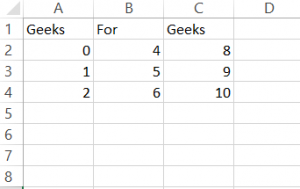geeksforgeeks2.csv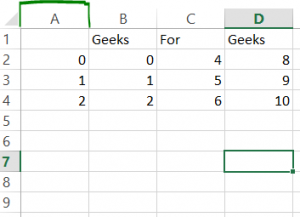```

2. Handling Missing Data

The Data Analysis Phase also comprises of the ability to handle the missing data from our dataset, and not so surprisingly Pandas live up to that expectation as well. This is where `dropna` and/or `fillna` methods comes into the play. While dealing with the missing data, you as a Data Analyst are either supposed to drop the column containing the NaN values (dropna method) or fill in the missing data with mean or mode of the whole column entry (fillna method), this decision is of great significance and depends upon the data and the affect would create in our results.

• Drop the missing Data :
Consider this is the DataFrame generated by below code :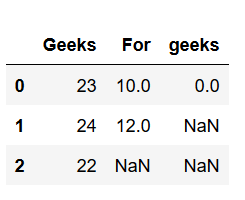`import` `pandas as pd`` ` `# Create a DataFrame``dframe ``=` `pd.DataFrame({``'Geeks'``: [``23``, ``24``, ``22``], ``                       ``'For'``: [``10``, ``12``, np.nan],``                       ``'geeks'``: [``0``, np.nan, np.nan]},``                       ``columns ``=``[``'Geeks'``, ``'For'``, ``'geeks'``])`` ` `# This will remove all the``# rows with NAN values`` ` `# If axis is not defined then``# it is along rows i.e. axis = 0``dframe.dropna(inplace ``=` `True``)``print``(dframe)`` ` `# if axis is equal to 1``dframe.dropna(axis ``=` `1``, inplace ``=` `True``)`` ` `print``(dframe)`

Output :

```axis=0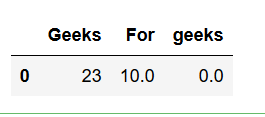axis=1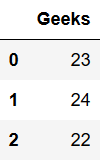```
•

• Fill the missing values :
Now, to replace any NaN value with mean or mode of the data, `fillna` is used, which could replace all the NaN values from a particular column or even in whole DataFrame as per the requirement.

 `import` `numpy as np``import` `pandas as pd`` ` `# Create a DataFrame``dframe ``=` `pd.DataFrame({``'Geeks'``: [``23``, ``24``, ``22``], ``                        ``'For'``: [``10``, ``12``, np.nan],``                        ``'geeks'``: [``0``, np.nan, np.nan]},``                        ``columns ``=` `[``'Geeks'``, ``'For'``, ``'geeks'``])`` ` `# Use fillna of complete Dataframe `` ` `# value function will be applied on every column``dframe.fillna(value ``=` `dframe.mean(), inplace ``=` `True``)``print``(dframe)`` ` `# filling value of one column``dframe[``'For'``].fillna(value ``=` `dframe[``'For'``].mean(),``                                    ``inplace ``=` `True``)``print``(dframe)`

Output :

`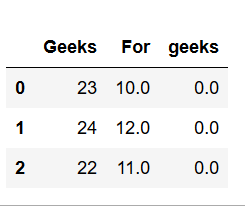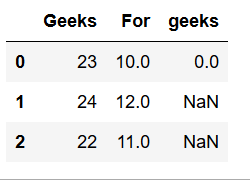`

3. Groupby Method (Aggregation) :

The groupby method allows us to group together the data based off any row or column, thus we can further apply the aggregate functions to analyze our data. Group series using mapper (dict or key function, apply given function to group, return result as series) or by a series of columns.

Consider this is the DataFrame generated by below code :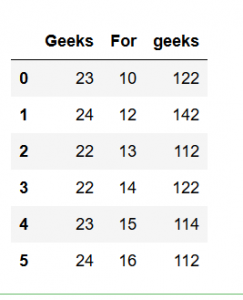`import` `pandas as pd``import` `numpy as np`` ` `# create DataFrame``dframe ``=` `pd.DataFrame({``'Geeks'``: [``23``, ``24``, ``22``, ``22``, ``23``, ``24``], ``                        ``'For'``: [``10``, ``12``, ``13``, ``14``, ``15``, ``16``],``                        ``'geeks'``: [``122``, ``142``, ``112``, ``122``, ``114``, ``112``]},``                        ``columns ``=` `[``'Geeks'``, ``'For'``, ``'geeks'``]) `` ` `# Apply groupby and aggregate function``# max to find max value of column `` ` `# "For" and column "geeks" for every``# different value of column "Geeks".`` ` `print``(dframe.groupby([``'Geeks'``]).``max``())`

Output :

```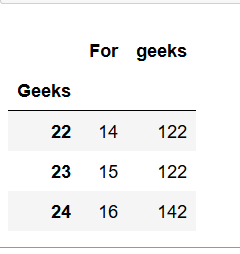```

My Personal Notes arrow_drop_up